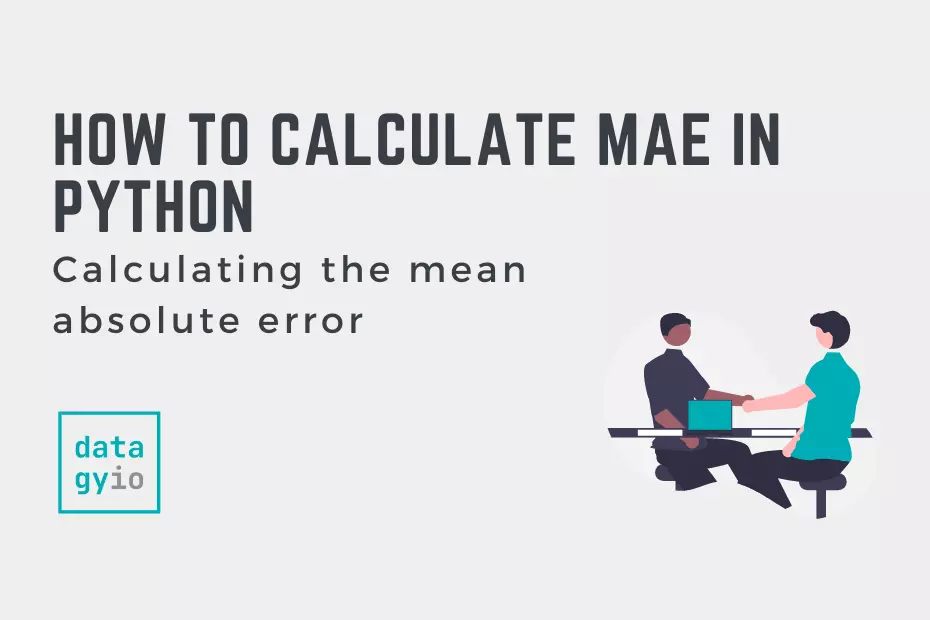# Error Statistics## How to Calculate Mean Absolute Error (MAE) in Python

In this tutorial, you’ll learn how to calculate the mean absolute error, or MAE, in Python. The mean absolute error can help measure the accuracy of a given machine learning model. The MAE can be a good complement or alternative to… Read More »How to Calculate Mean Absolute Error (MAE) in Python## How to Calculate MAPE in Python

In this tutorial, you’ll learn how to use Python to calculate the MAPE, or the mean absolute percentage error. This error is often used to measure the accuracy of machine learning models. By the end of this tutorial, you’ll have… Read More »How to Calculate MAPE in Python